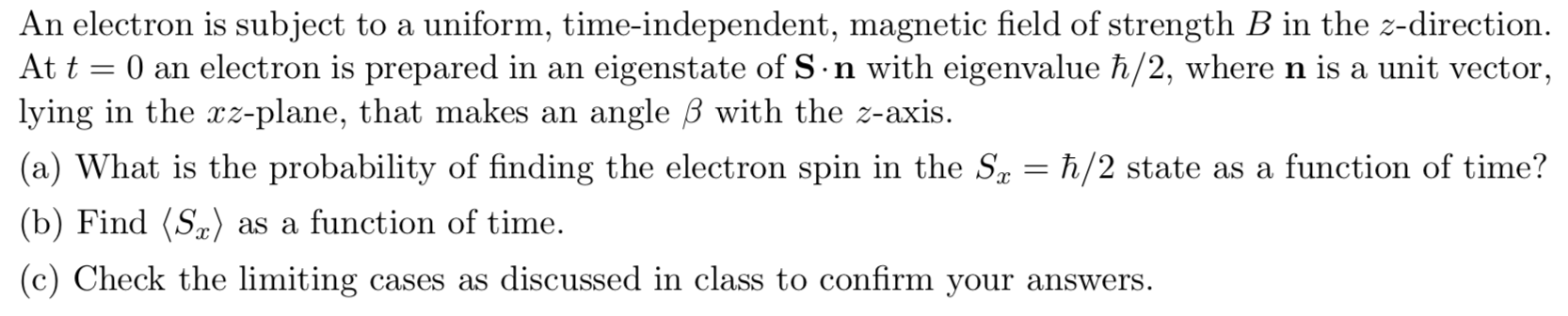# An electron is subject to a uniform, time-independent, magnetic field of strength B in the z-directionAt t 0 an electron is prepared in anlying in the rz-plane, that makes aneigenstate of S n with eigenvalue h/2, wheren is a unit vectorangle B with the z-axis.(a) What is the probability of finding the electron spin in the Sa = h/2 state as a function of time?(b) Find (Sa)as a function of time(c) Check the limitingcases as discussed in class to confirm your answers.

Question
150 views

It's a quantum mechanics question.help_outlineImage TranscriptioncloseAn electron is subject to a uniform, time-independent, magnetic field of strength B in the z-direction At t 0 an electron is prepared in an lying in the rz-plane, that makes an eigenstate of S n with eigenvalue h/2, wheren is a unit vector angle B with the z-axis. (a) What is the probability of finding the electron spin in the Sa = h/2 state as a function of time? (b) Find (Sa) as a function of time (c) Check the limiting cases as discussed in class to confirm your answers. fullscreen
check_circle

star
star
star
star
star
1 Rating
Step 1

The unit vector n and the spin vector S can be represented in the xz plane as,

Step 2

In the second equation above σ is the representation of the pauli spin vector and θ is the angle between the direction of the Spin angular momentum vector S and the z axis.

Hence the dot product of the spin angular momentum S vector and the unit vector n can be represented as,

Step 3

Let |ψ> denote an eigenstate of the operat...

### Want to see the full answer?

See Solution

#### Want to see this answer and more?

Solutions are written by subject experts who are available 24/7. Questions are typically answered within 1 hour.*

See Solution
*Response times may vary by subject and question.
Tagged in

### Science